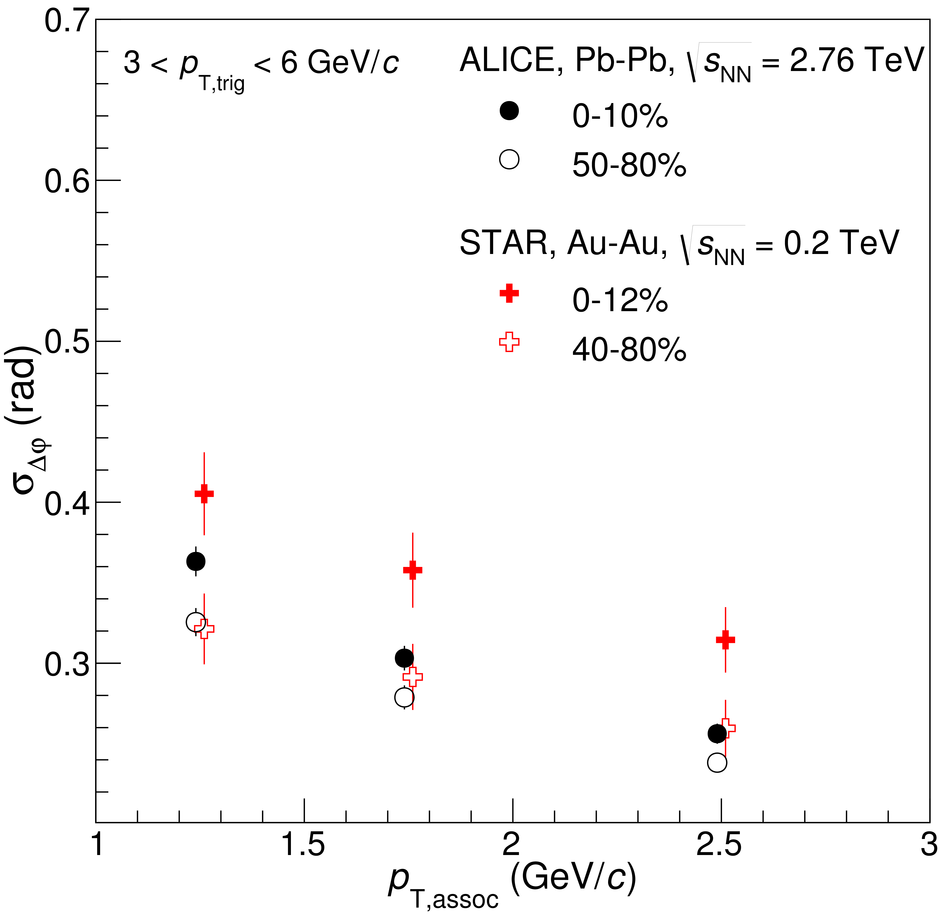# Figure 10

 Comparison of the shape parameters $\sigma_{\Dphi}$ (left panel) and $\sigma_{\Deta}$ (right panel) to results from the STAR collaboration in Au--Au collisions at $\snn = \unit{GeV}$~ The markers are placed at the centre of the $p_{\rm T,assoc}$ bins, slightly displaced for visibility, and the uncertainties are statistical and systematic ones added in quadrature